# Regular Expressions Chapter 6 1 Regular Languages L

• Slides: 62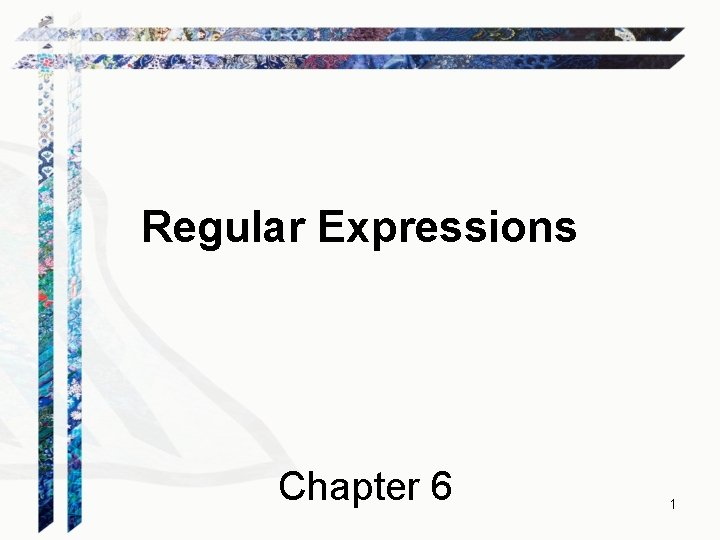Regular Expressions Chapter 6 1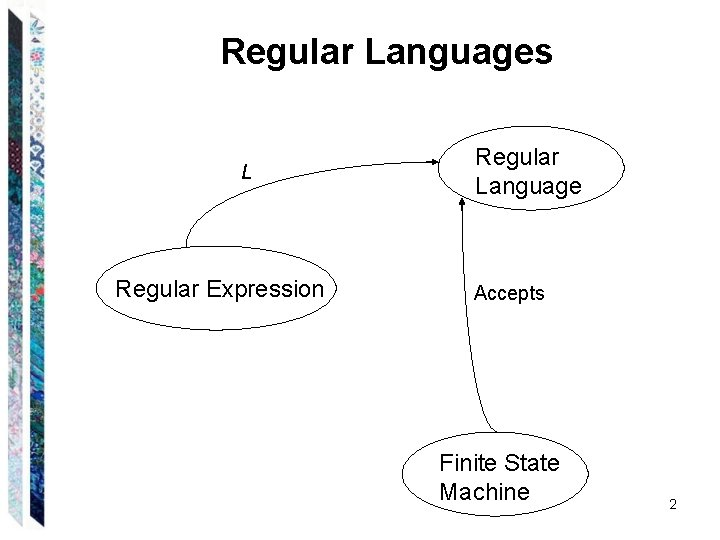Regular Languages L Regular Expression Regular Language Accepts Finite State Machine 2Regular Expressions The regular expressions over an alphabet are all and only the strings that can be obtained as follows: 1. is a regular expression. 2. is a regular expression. 3. Every element of is a regular expression. 4. If , are regular expressions, then so is . 5. If , are regular expressions, then so is . 6. If is a regular expression, then so is *. 7. is a regular expression, then so is +. 8. If is a regular expression, then so is ( ). 3Regular Expression Examples If = {a, b}, the following are regular expressions: a (a b)* abba 4Regular Expressions Define Languages Define L, a semantic interpretation function for regular expressions: 1. L( ) = . 2. L( ) = { }. 3. L(c), where c = {c}. 4. L( ) = L( ). 5. L( ) = L( ). 6. L( *) = (L( ))*. 7. L( +) = L( *) = L( ) (L( ))*. If L( ) is equal to , then L( +) is also equal to . Otherwise L( +) is the language that is formed by concatenating together one or more strings drawn from L( ). 8. L(( )) = L( ). 5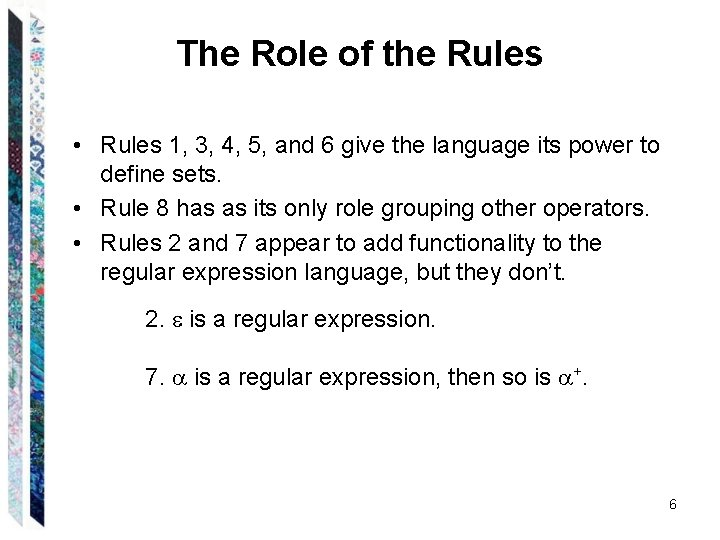The Role of the Rules • Rules 1, 3, 4, 5, and 6 give the language its power to define sets. • Rule 8 has as its only role grouping other operators. • Rules 2 and 7 appear to add functionality to the regular expression language, but they don’t. 2. is a regular expression. 7. is a regular expression, then so is +. 6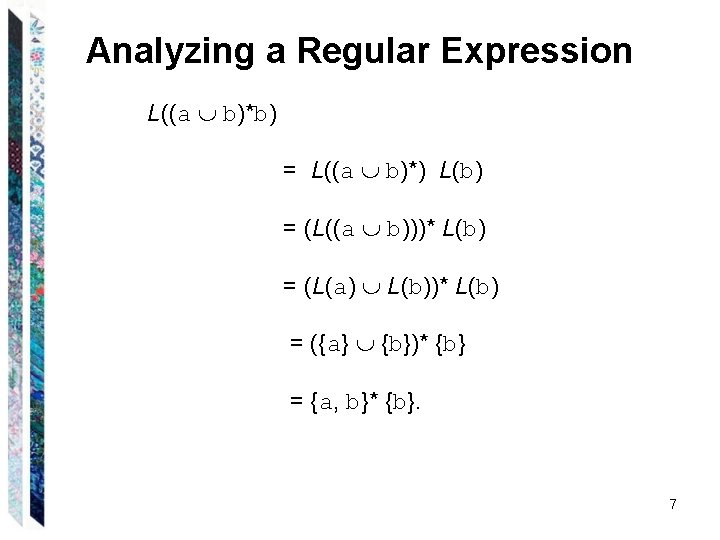Analyzing a Regular Expression L((a b)*b) = L((a b)*) L(b) = (L((a b)))* L(b) = (L(a) L(b))* L(b) = ({a} {b})* {b} = {a, b}* {b}. 7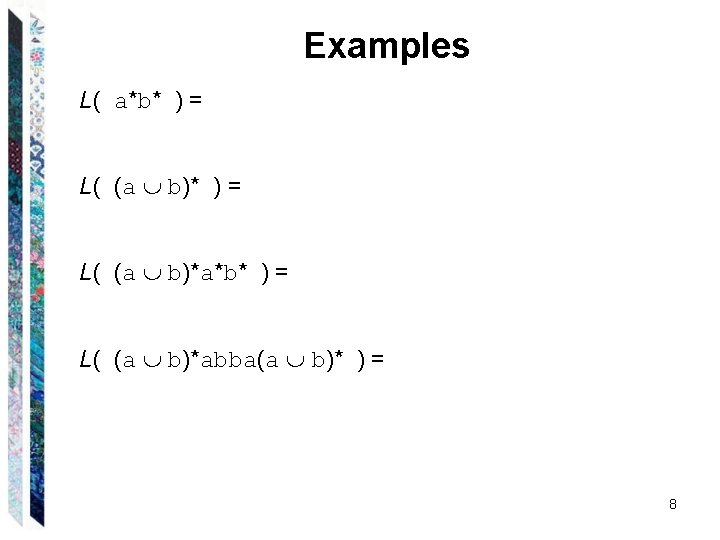Examples L( a*b* ) = L( (a b)*a*b* ) = L( (a b)*abba(a b)* ) = 8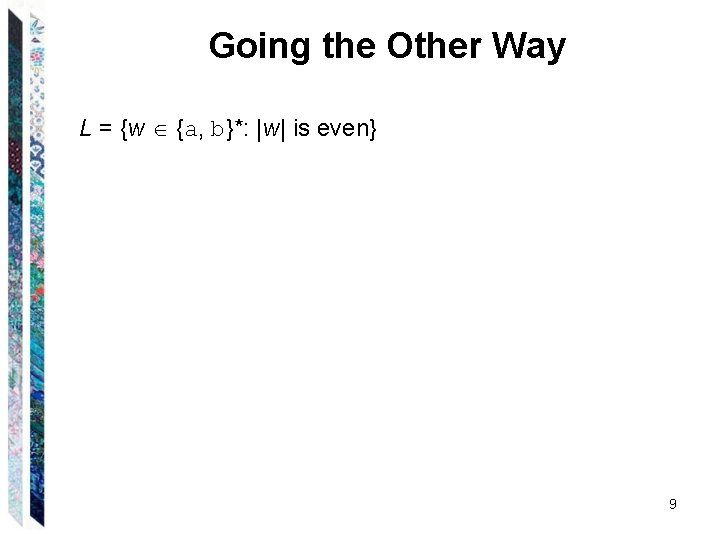Going the Other Way L = {w {a, b}*: |w| is even} 9Going the Other Way L = {w {a, b}*: |w| is even} ((a b))* (aa ab ba bb)* 10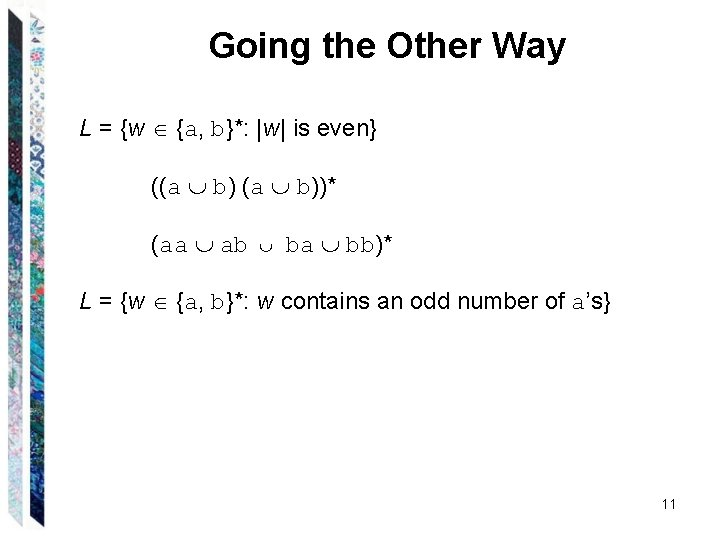Going the Other Way L = {w {a, b}*: |w| is even} ((a b))* (aa ab ba bb)* L = {w {a, b}*: w contains an odd number of a’s} 11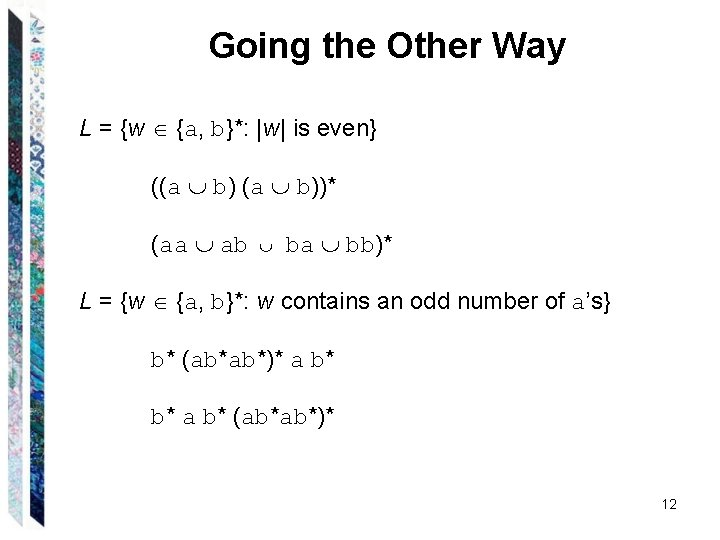Going the Other Way L = {w {a, b}*: |w| is even} ((a b))* (aa ab ba bb)* L = {w {a, b}*: w contains an odd number of a’s} b* (ab*ab*)* a b* b* a b* (ab*ab*)* 12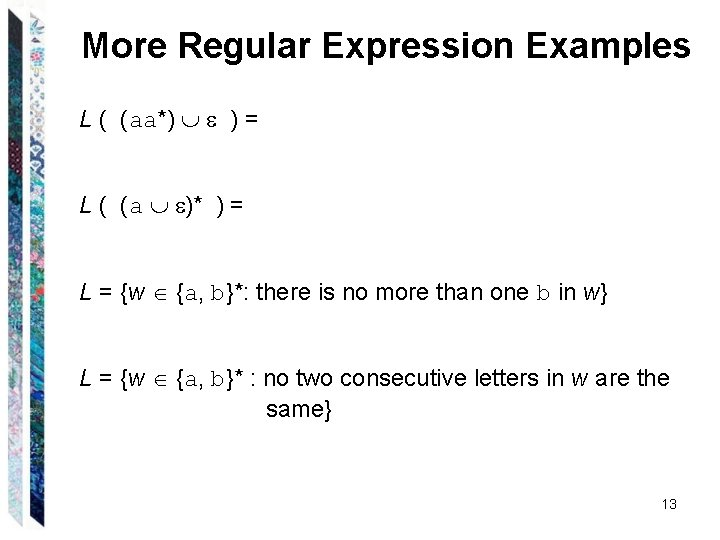More Regular Expression Examples L ( (aa*) ) = L ( (a )* ) = L = {w {a, b}*: there is no more than one b in w} L = {w {a, b}* : no two consecutive letters in w are the same} 13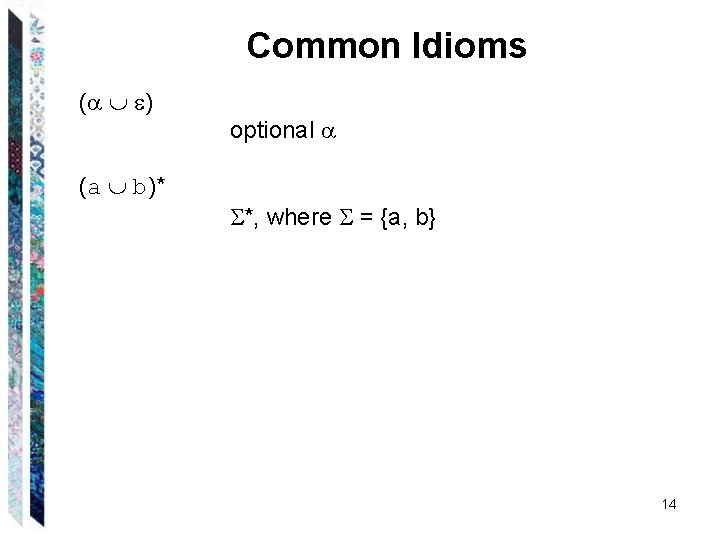Common Idioms ( ) (a b)* optional *, where = {a, b} 14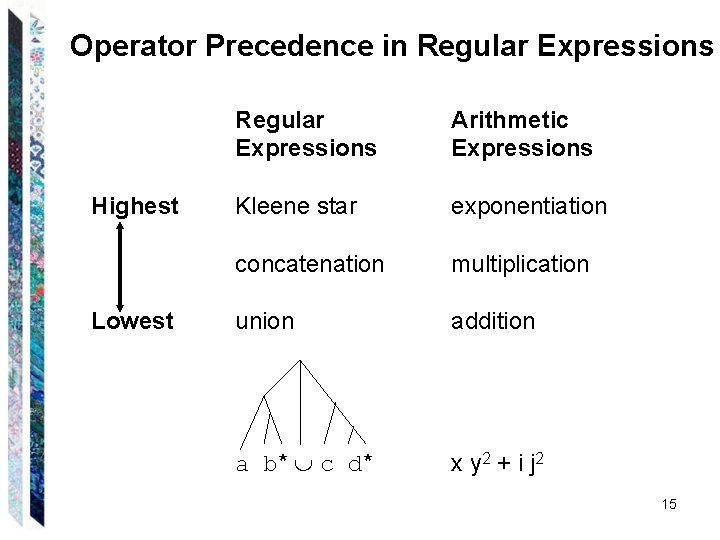Operator Precedence in Regular Expressions Highest Lowest Regular Expressions Arithmetic Expressions Kleene star exponentiation concatenation multiplication union addition a b* c d* x y 2 + i j 2 15The Details Matter a* b* (a b)* (ab)* a*b* 16Kleene’s Theorem Finite state machines and regular expressions define the same class of languages. To prove this, we must show: To prove A = B, we have to prove: 1. A B and 2. B A Theorem: Any language that can be defined with a regular expression can be accepted by some FSM and so is regular. Theorem: Every regular language (i. e. , every language that can be accepted by some DFSM) can be defined with a regular expression. 17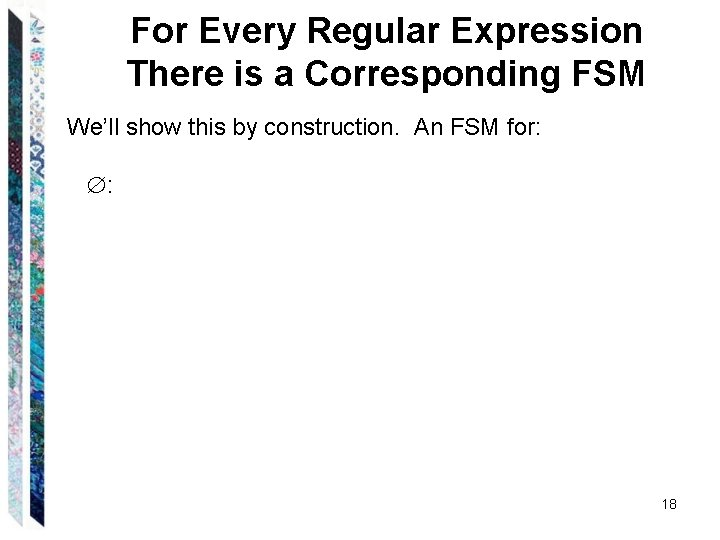For Every Regular Expression There is a Corresponding FSM We’ll show this by construction. An FSM for: : 18For Every Regular Expression There is a Corresponding FSM We’ll show this by construction. An FSM for: : 19For Every Regular Expression There is a Corresponding FSM We’ll show this by construction. An FSM for: : A single element c of : 20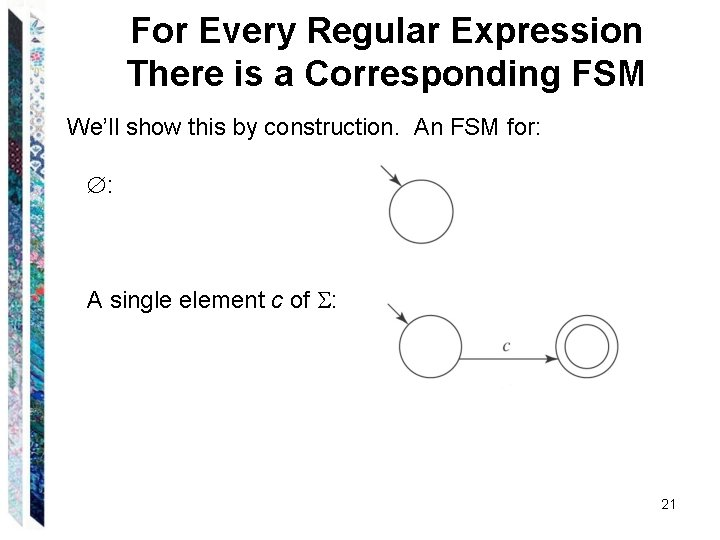For Every Regular Expression There is a Corresponding FSM We’ll show this by construction. An FSM for: : A single element c of : 21For Every Regular Expression There is a Corresponding FSM We’ll show this by construction. An FSM for: : A single element c of : ( *): 22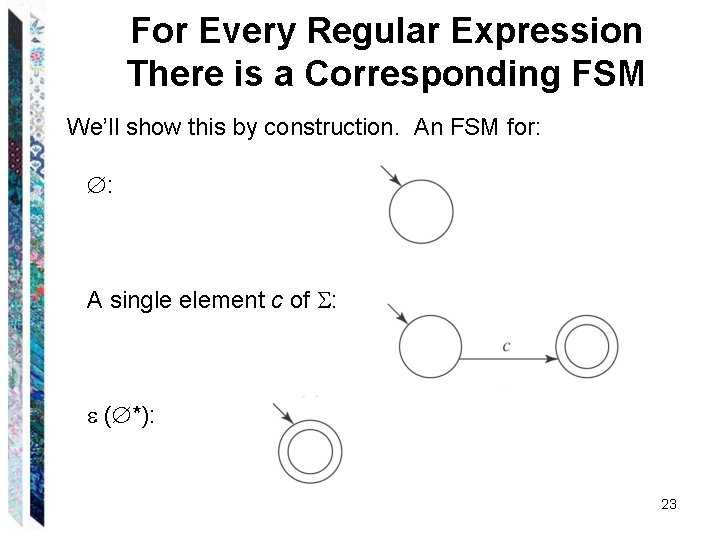For Every Regular Expression There is a Corresponding FSM We’ll show this by construction. An FSM for: : A single element c of : ( *): 23Union If is the regular expression and if both L( ) and L( ) are regular: 24Union S 3 25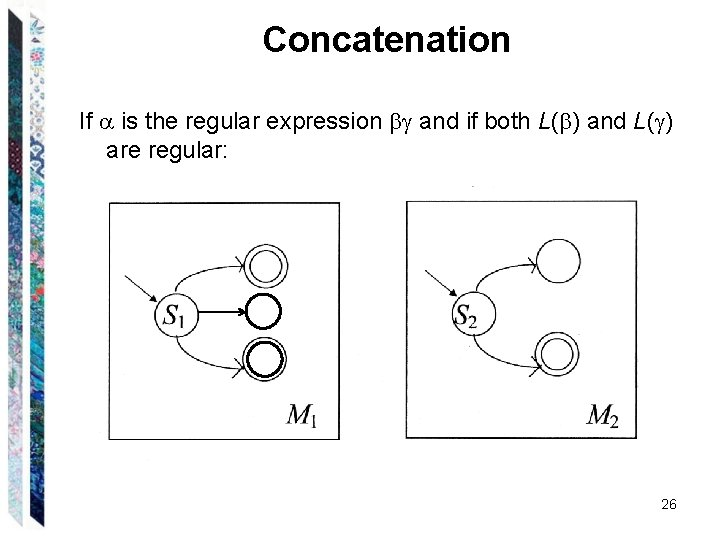Concatenation If is the regular expression and if both L( ) and L( ) are regular: 26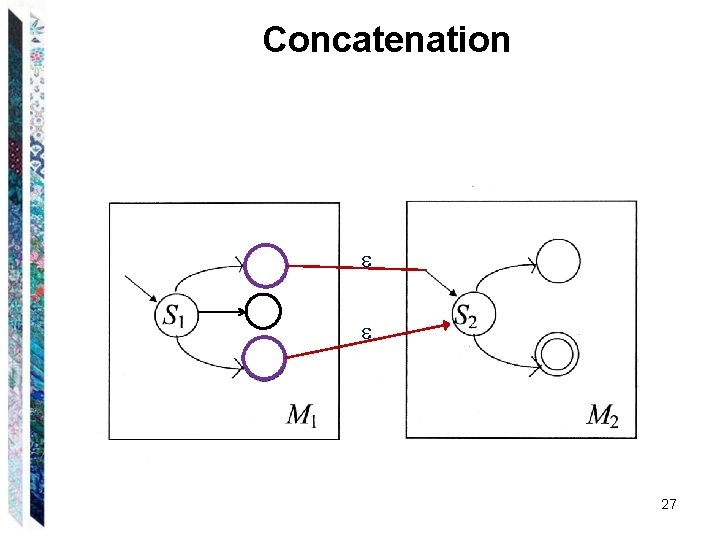Concatenation 27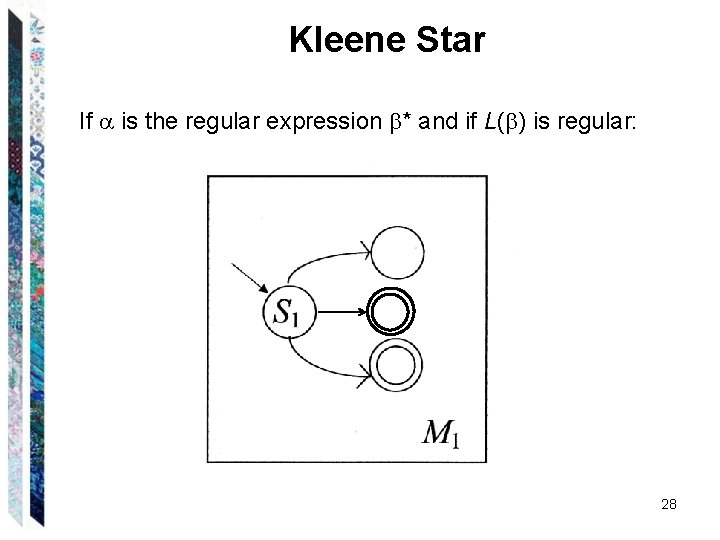Kleene Star If is the regular expression * and if L( ) is regular: 28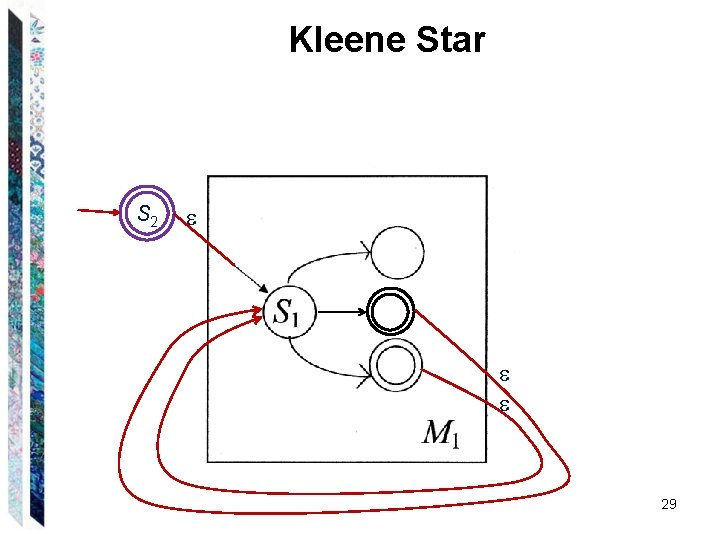Kleene Star S 2 29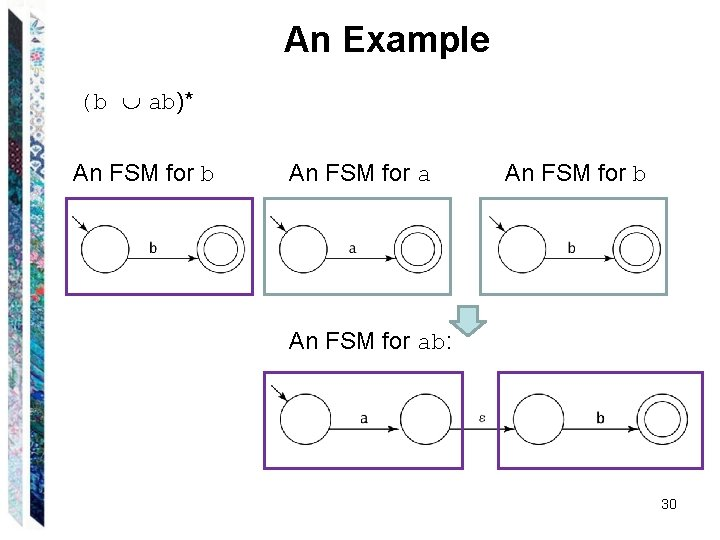An Example (b ab)* An FSM for b An FSM for ab: 30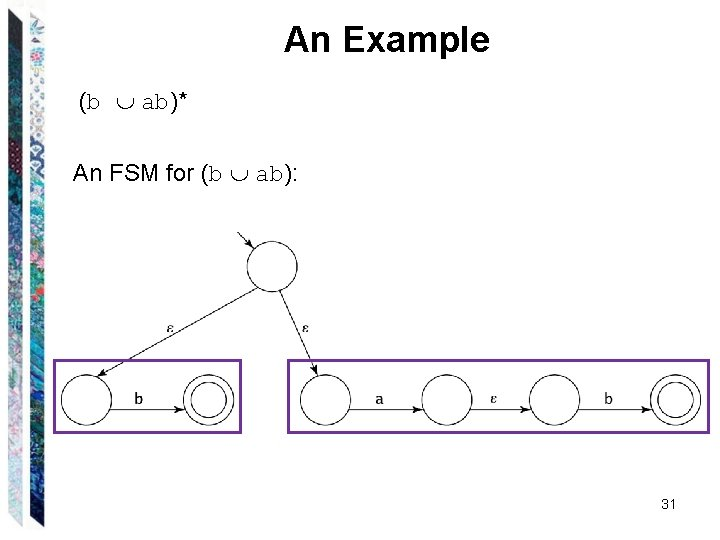An Example (b ab)* An FSM for (b ab): 31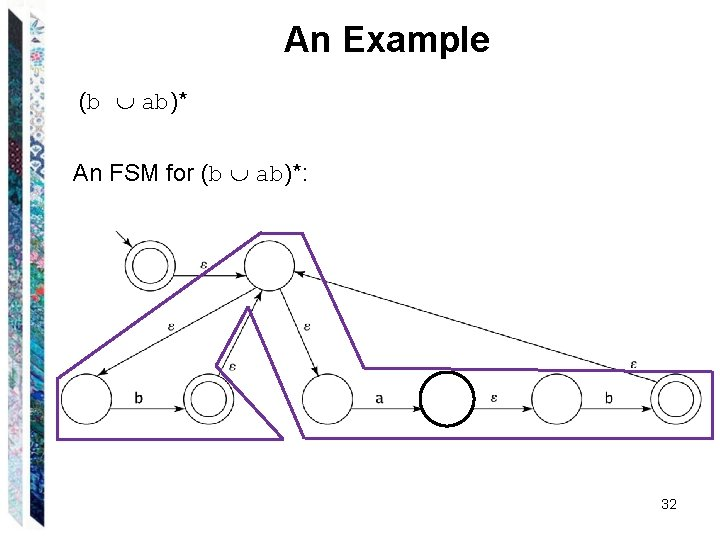An Example (b ab)* An FSM for (b ab)*: 32The Algorithm regextofsm( : regular expression) = Beginning with the primitive subexpressions of and working outwards until an FSM for all of has been built do: Construct an FSM as described above. 33For Every FSM There is a Corresponding Regular Expression We’ll show this by construction. The key idea is that we’ll allow arbitrary regular expressions to label the transitions of an FSM. 34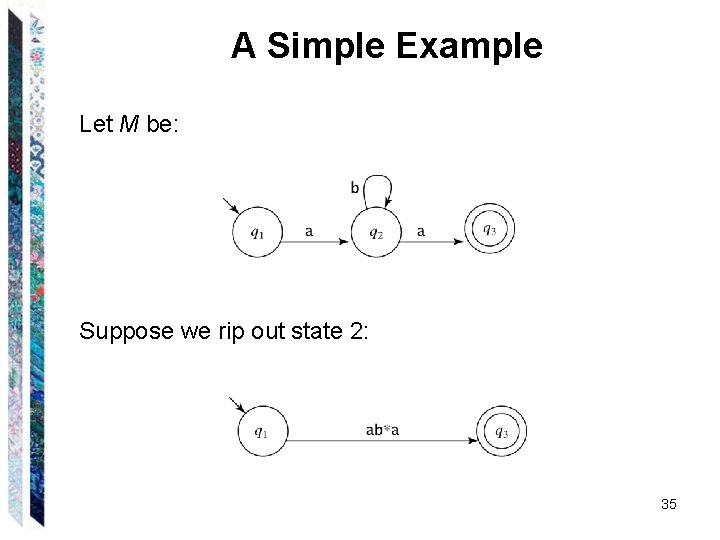A Simple Example Let M be: Suppose we rip out state 2: 35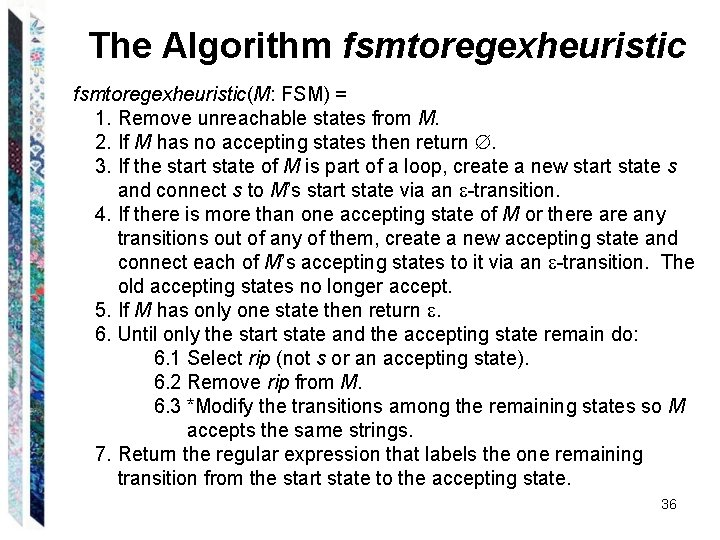The Algorithm fsmtoregexheuristic(M: FSM) = 1. Remove unreachable states from M. 2. If M has no accepting states then return . 3. If the start state of M is part of a loop, create a new start state s and connect s to M’s start state via an -transition. 4. If there is more than one accepting state of M or there any transitions out of any of them, create a new accepting state and connect each of M’s accepting states to it via an -transition. The old accepting states no longer accept. 5. If M has only one state then return . 6. Until only the start state and the accepting state remain do: 6. 1 Select rip (not s or an accepting state). 6. 2 Remove rip from M. 6. 3 *Modify the transitions among the remaining states so M accepts the same strings. 7. Return the regular expression that labels the one remaining transition from the start state to the accepting state. 36An Example 1. Create a new initial state and a new, unique accepting state, neither of which is part of a loop. 37An Example, Continued It’s to create a source and a sink! 2. Remove states and arcs and replace with arcs labelled with larger and larger regular expressions. 38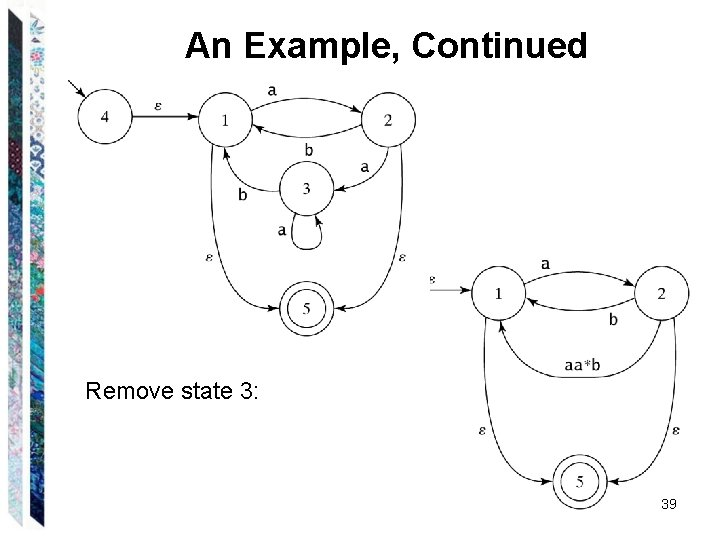An Example, Continued Remove state 3: 39An Example, Continued Remove state 2: 40An Example, Continued The goal is to keep the source and the sink only! Remove state 1: 41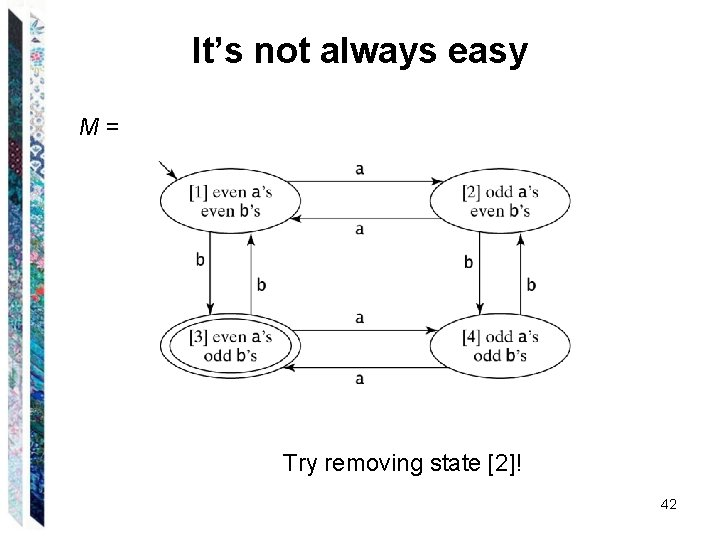It’s not always easy M= Try removing state ! 42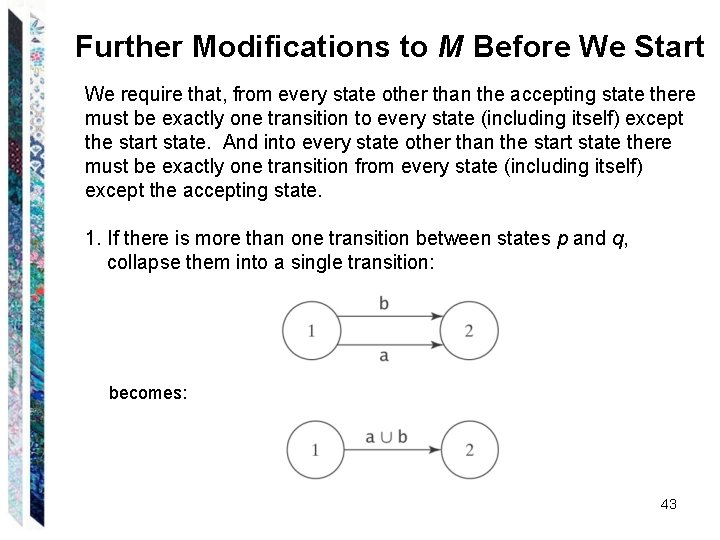Further Modifications to M Before We Start We require that, from every state other than the accepting state there must be exactly one transition to every state (including itself) except the start state. And into every state other than the start state there must be exactly one transition from every state (including itself) except the accepting state. 1. If there is more than one transition between states p and q, collapse them into a single transition: becomes: 43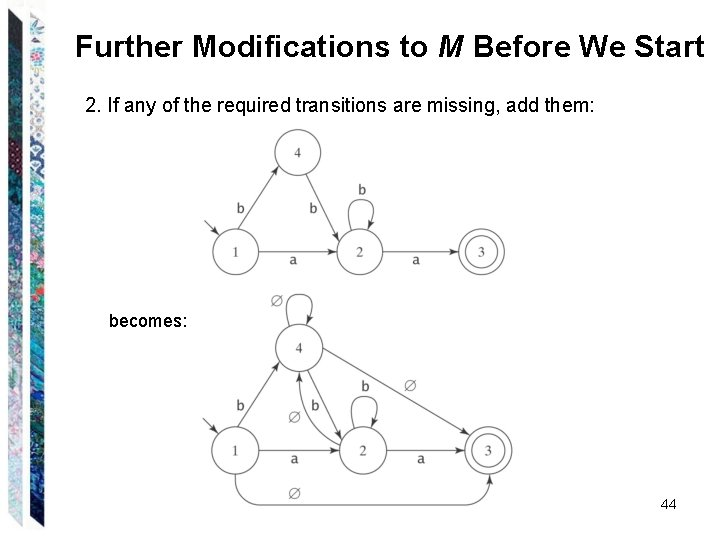Further Modifications to M Before We Start 2. If any of the required transitions are missing, add them: becomes: 44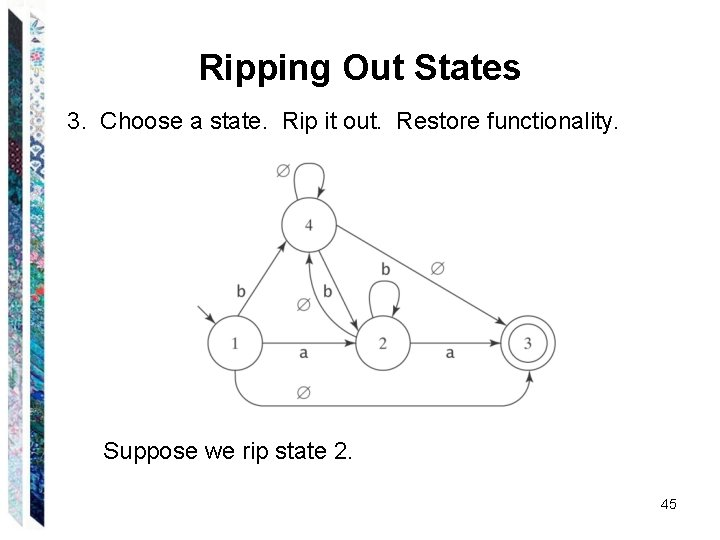Ripping Out States 3. Choose a state. Rip it out. Restore functionality. Suppose we rip state 2. 45What Happens When We Rip? Consider any pair of states p and q. Once we remove rip, how can M get from p to q? ● It can still take the transition that went directly from p to q, or ● It can take the transition from p to rip. Then, it can take the transition from rip back to itself zero or more times. Then it can take the transition from rip to q. 46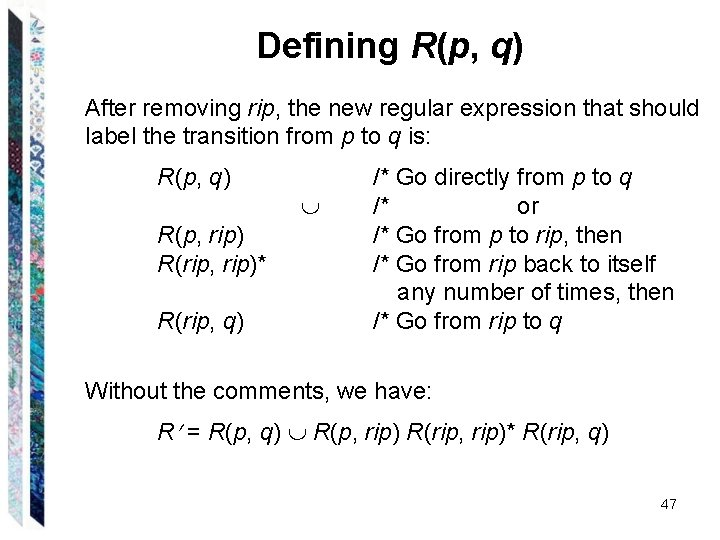Defining R(p, q) After removing rip, the new regular expression that should label the transition from p to q is: R(p, q) R(p, rip) R(rip, rip)* R(rip, q) /* Go directly from p to q /* or /* Go from p to rip, then /* Go from rip back to itself any number of times, then /* Go from rip to q Without the comments, we have: R = R(p, q) R(p, rip) R(rip, rip)* R(rip, q) 47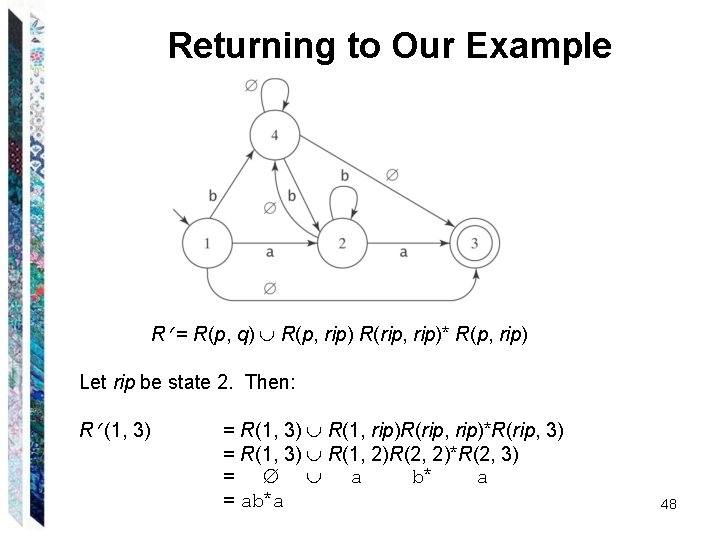Returning to Our Example R = R(p, q) R(p, rip) R(rip, rip)* R(p, rip) Let rip be state 2. Then: R (1, 3) = R(1, 3) R(1, rip)R(rip, rip)*R(rip, 3) = R(1, 3) R(1, 2)R(2, 2)*R(2, 3) = a b* a = ab*a 48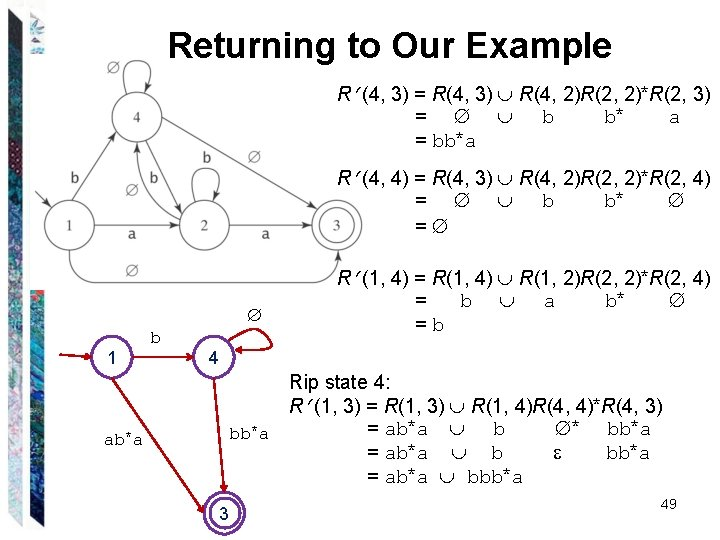Returning to Our Example R (4, 3) = R(4, 3) R(4, 2)R(2, 2)*R(2, 3) = b b* a = bb*a R (4, 4) = R(4, 3) R(4, 2)R(2, 2)*R(2, 4) = b b* = 1 ab*a b R (1, 4) = R(1, 4) R(1, 2)R(2, 2)*R(2, 4) = b a b* =b 4 bb*a 3 Rip state 4: R (1, 3) = R(1, 3) R(1, 4)R(4, 4)*R(4, 3) = ab*a b * bb*a = ab*a bbb*a 49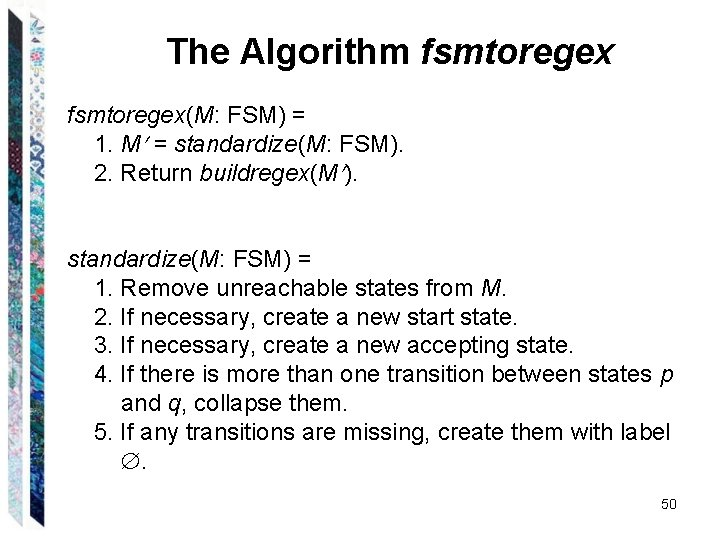The Algorithm fsmtoregex(M: FSM) = 1. M = standardize(M: FSM). 2. Return buildregex(M ). standardize(M: FSM) = 1. Remove unreachable states from M. 2. If necessary, create a new start state. 3. If necessary, create a new accepting state. 4. If there is more than one transition between states p and q, collapse them. 5. If any transitions are missing, create them with label . 50The Algorithm fsmtoregex buildregex(M: FSM) = 1. If M has no accepting states then return . 2. If M has only one state, then return . 3. Until only the start and accepting states remain do: 3. 1 Select some state rip of M. 3. 2 For every transition from p to q, if both p and q are not rip then do Compute the new label R for the transition The case of p = q should from p to q: also be considered! R (p, q) = R(p, q) R(p, rip) R(rip, rip)* R(rip, q) 3. 3 Remove rip and all transitions into and out of it. 4. Return the regular expression that labels the transition from the start state to the accepting state. 51Regular Expression or FSM • Kleene’s Theorem – Regular expression FSM • Q: when to use regular expression and when to use FSM to describe a regular language? • Order – Regular expression: must specify the order in which a sequence of symbols must occur • Phone number, email address, etc. – FSM: order doesn’t matter • Vending machine, parity checking, etc. • Sometimes it’s easier to do it one way, sometimes the other. 52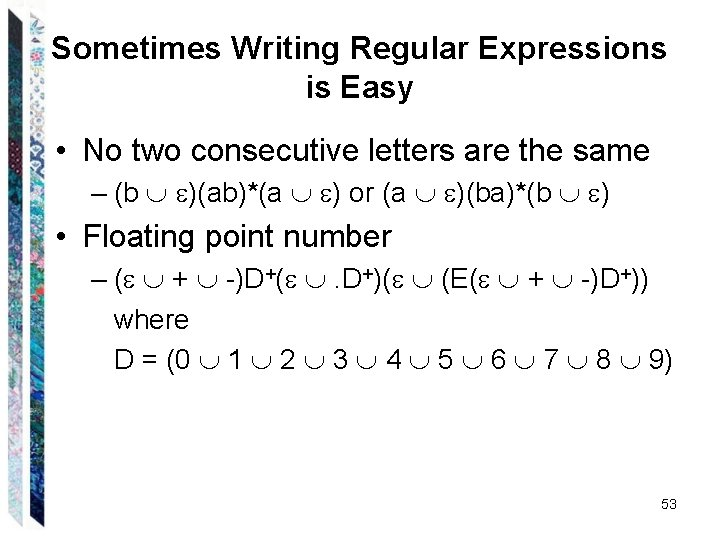Sometimes Writing Regular Expressions is Easy • No two consecutive letters are the same – (b )(ab)*(a ) or (a )(ba)*(b ) • Floating point number – ( + -)D+( . D+)( (E( + -)D+)) where D = (0 1 2 3 4 5 6 7 8 9) 53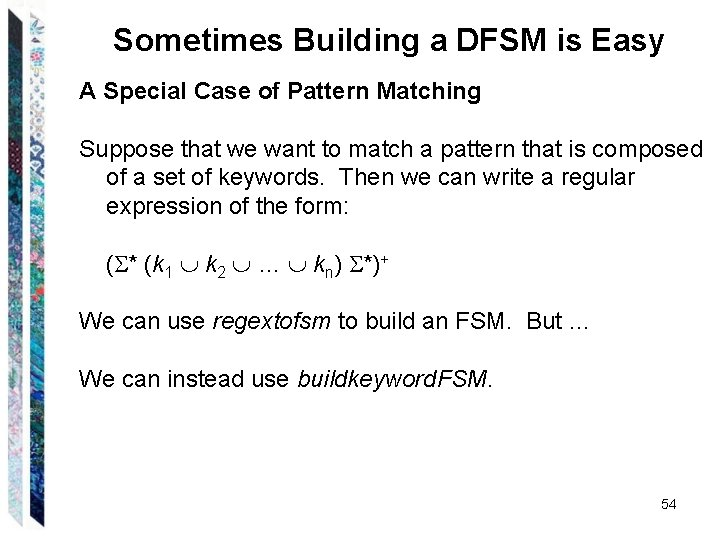Sometimes Building a DFSM is Easy A Special Case of Pattern Matching Suppose that we want to match a pattern that is composed of a set of keywords. Then we can write a regular expression of the form: ( * (k 1 k 2 … kn) *)+ We can use regextofsm to build an FSM. But … We can instead use buildkeyword. FSM. 54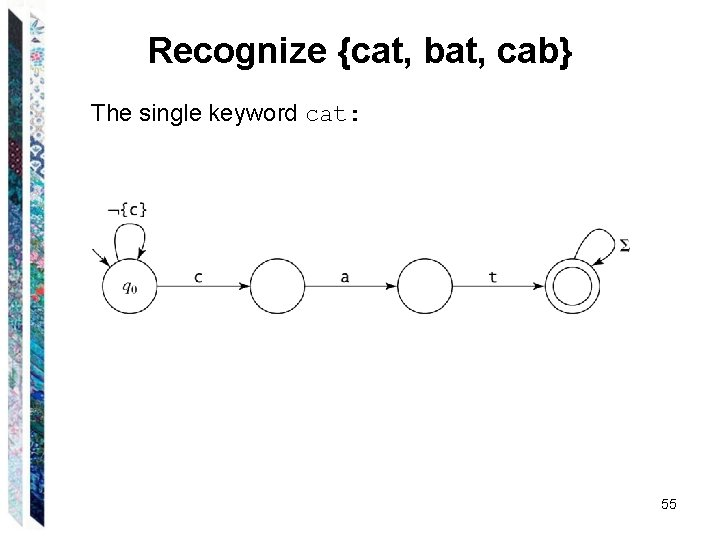Recognize {cat, bat, cab} The single keyword cat: 55{cat, bat, cab} Adding bat and cab: 56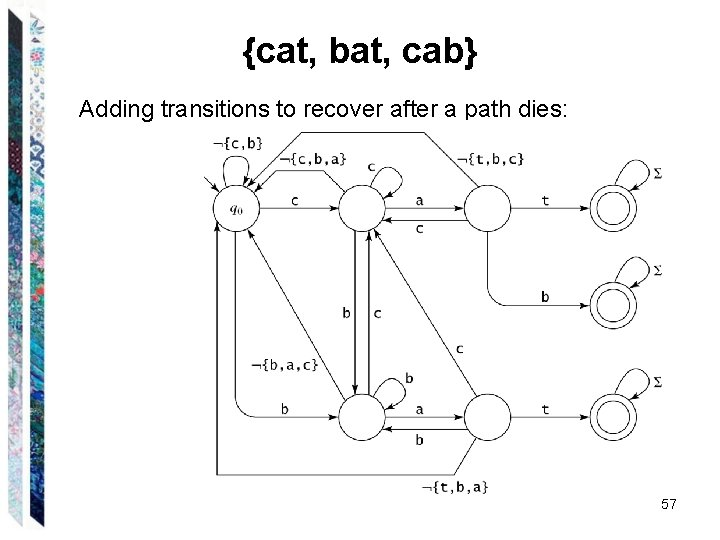{cat, bat, cab} Adding transitions to recover after a path dies: 57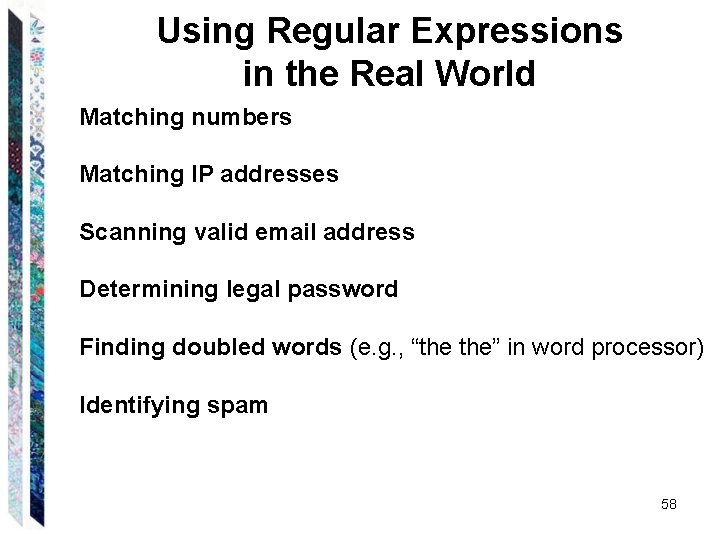Using Regular Expressions in the Real World Matching numbers Matching IP addresses Scanning valid email address Determining legal password Finding doubled words (e. g. , “the the” in word processor) Identifying spam 58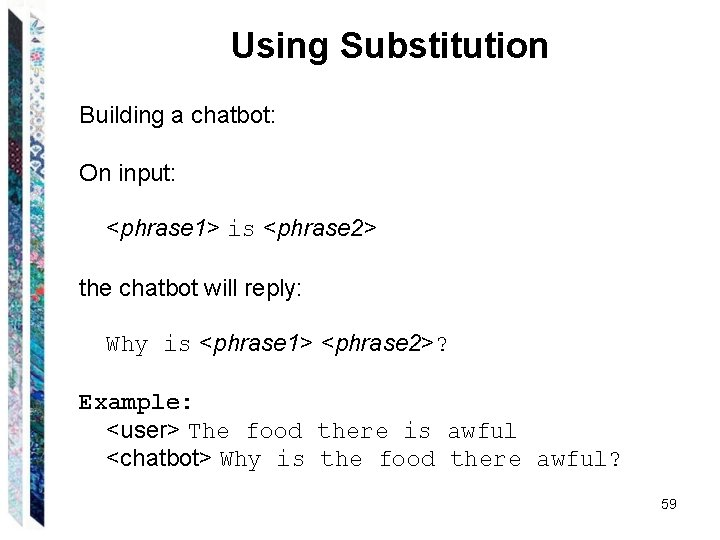Using Substitution Building a chatbot: On input: <phrase 1> is <phrase 2> the chatbot will reply: Why is <phrase 1> <phrase 2>? Example: <user> The food there is awful <chatbot> Why is the food there awful? 59Simplifying Regular Expressions Regular expression as sets: ● Union is commutative: = ● Union is associative: ( ) = ( ) ● is the identity for union: = = ● Union is idempotent: = ● If B A, A B = A Concatenation: ● Concatenation is associative: ( ) = ( ) ● is the identity for concatenation: = = ● is a zero for concatenation: = = Concatenation distributes over union: ● ( ) = ( ) ● ( ) = ( ) 60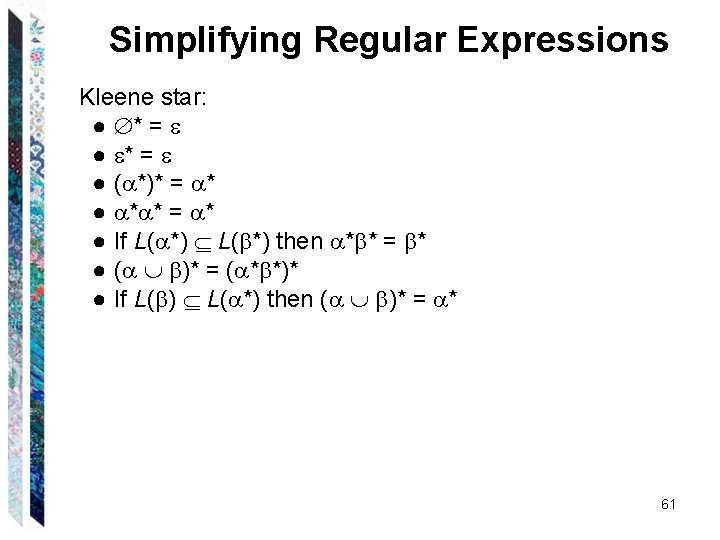Simplifying Regular Expressions Kleene star: ● * = ● ( *)* = * ● * * = * ● If L( *) then * * = * ● ( )* = ( * *)* ● If L( ) L( *) then ( )* = * 61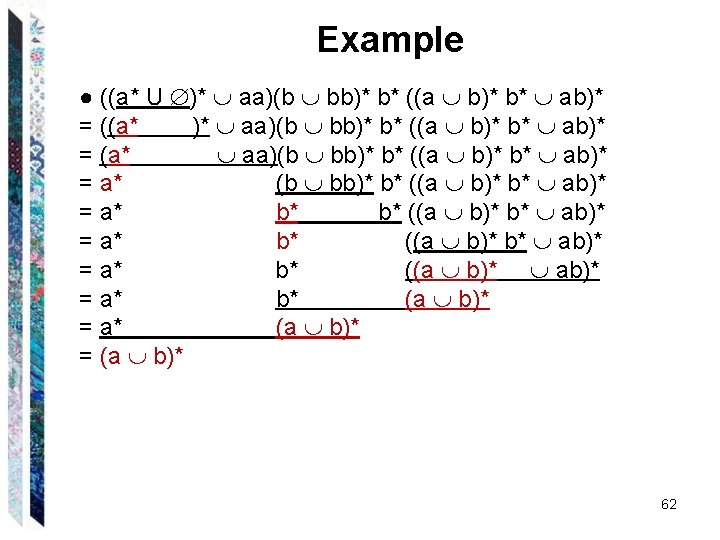Example ● ((a* U )* aa)(b bb)* b* ((a b)* b* ab)* = ((a* )* aa)(b bb)* b* ((a b)* b* ab)* = (a* aa)(b bb)* b* ((a b)* b* ab)* = a* b* ((a b)* ab)* = a* b* (a b)* = a* (a b)* = (a b)* 62